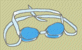# Natavision

### Stopwatch Calculator for Android

A calculator for stopwatch arithmetic. Add, subtract, multiply, and divide race times without converting to seconds. Perfect for swimming and running / track / cross county. Intended for coaches, parents, and athletes.

Times are in HH:mm:ss.hh format (hours, minutes, seconds, hundredths). There is no need to type the colon or period, so "12345" is interpreted as "1:23.45" and ":30" is interpreted as "30.00".

Examples:

1. Calculating splits. Leg 4 started at 3:14.67 and ended at 4:11.50. What was leg 4's split?

4 1 1 5 0 - 3 1 4 6 7 = 56.83

2. Calculating pace. Runner A finished the marathon in 3:26:54.48. What was her mile pace?

3 2 6 5 4 4 8 ÷ 2 6 . 2 = 7:53.83

3. Calculating set duration. How long will 20 50s on :55 take to swim in practice?

: 5 5 × 2 0 = 18:20.00

4. Calculating relay seed times. The four runners' individual times are 54.68, 57.32, 57.98, and 1:00.04. What would their relay time be?

5 4 6 8 + 5 7 3 2 + 5 7 9 8 + 1 0 0 0 4 = 3:50.02

5. Converting between courses. Swimmer A swam a 5:44.86 short course yards 400 IM. What is that in meters? (1 yard = 0.9144 meters)

5 4 4 8 6 × . 9 1 4 4 = 5:15.33

Runs on Android phones and tablets. Available on Google Play.